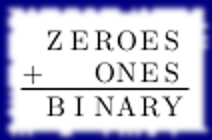# Use Math Puzzles To Start A Dialogue

If you’ve been following along for the last few days either on the blog or on Twitter, you’ll have seen the alphanumeric puzzles I’ve put up. Here is the first puzzleFor other puzzles, just type in “alphanumeric” in the blog search [it’s in different locations, depending on what device you’re on].

One of the things I like about these puzzles is not necessarily solving them, but in constructing them. Both acts of doing (making and solving) have math value.

From a maker’s perspective, one of the things I try to do is to find a reasonable balance between word lengths, puzzle difficulty (when done by hand), and a thematic connection. The thematic connection, I would say, is more qualitative than quantitative. Puzzle difficulty, which is a function of word lengths and operator choices, at minimum, is not something that I’ve quantified just yet, but I’m on my way to standardizing a scoring process.

So from the standpoint of math education value in making such puzzles, I do have to think about and understand how numbers interact with each other through operations ($$+, -, \times, \div$$). But before I head down the route of figuring out what numbers can work, I want to know what possible structures can work.

To that end, here are some questions you can ask your students or maybe ponder for yourself about puzzle structure. This is a good way to at least start the dialogue in your classroom or home and it is also a good way to bring some context to the arithmetic that we learn / teach. Remember context doesn’t have to be grounded in the hard sciences. Context can be in the construction of a game, or in this case, a puzzle!

• What’s the maximum and minimum number of digits that can be obtained by adding two 3-digit numbers? (Generally $$k$$-digit numbers.) (And even more generally, what’s the maximum and minimum number of digits that can be obtained by adding $$N$$, $$k$$-digit numbers?)
• What’s the maximum and minimum number of digits that can be obtained by multiply a two-digit number, one-digit number, and a one-digit number?
• From the above two questions, we can then think about how numbers should interact. For example, even if structurally $$\mbox{BASE } + \mbox{BALL } = \mbox{GAME}$$ works, why can we tell that there won’t be any solution to this under the usual rules [one letter per number, no leading zeros, etc.]?
• Why would there be no solutions to this alpha numeric puzzle? $$PI \times RADIUS \times RADIUS = AREA$$
• Why might we want to investigate the possibility of a solution to $$PI \times R \times R = AREA$$
• What are other possible formulas you could construct that might have a chance of producing solutions?

I actually like the last question a lot because it sneaks in a review of formulas without the odiousness of “memorize these formulas”. Part of fluency is repetition and this type of repetition is a good kind, in my opinion. Of course, I’m not saying that this is how students should learn formulas, I’m saying that this is a minor, bonus side effect of thematic puzzle making. In any case, math play like this can make for fun side projects (even for older students, especially if you want them to learn to program) and if you’re able to get the discussion going, you’ll see that a lot more questions will emerge! How do I know if a puzzle will have a unique answer? How can I make puzzles more efficiently? How would I program a solver? And so on!

Do you want a puzzle created? Just ask either in the comment below, or by tweeting to me, or by sending me an email!